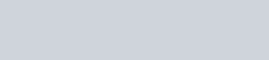Definition of Graham's Law and Explanations of Thermal Agitations - GulpMatrix

# Definition of Graham’s Law and Explanations of Thermal AgitationsWhat are Thermal Agitations? When a gas passes through a porous plug, a cotton-wool wad, for example, it is said to diffuse’. In that it is not a motion of the gas in bulk, but it a result of the motion of its individual molecules.

Graham (1805 – 69) devised an apparatus to compare the rates of diffusion of different gases. D is a glass tube, closed with a plug P of plaster of Paris. It is first filled with mercury, and inverted over mercury in a bowl. Hydrogen is then passed into it until the mercury levels are the same on each side; the hydrogen is then at atmospheric pressure. The volume of hydrogen, VH, is proportional to the length of the tube above the mercury. The apparatus is now left; hydrogen diffuses out through remains in the tube D. the tube is then adjusted until the level of mercury is again the same on each side, so that the air within it is again the same on each side, so that the air within it is at atmospheric pressure. The volume of air, VA, is proportional to the new length of the tube above the mercury.

The volumes VA and VH are, respectively, the volumes of air and hydrogen which diffused through the plug in the air and hydrogen which diffused through the plug in the same time. Therefore the rates of diffusion of the gases, air and hydrogen are proportional to the volumes VA and VH:And in words: the rate of diffusion of a gas is inversely proportional to the square root of its density. This is Graham’s Law. Graham’s law of diffusion is readily explained by the kinetic theory. At the same Kelvin temperature T, the mean kinetic energies of the molecules of different gases are equal, since.And k is a universal constant. Therefore, if the subscripts A and H denote air and hydrogen respectively,The average speed of the molecules of a gas is roughly equal to and strictly proportional to – the square root of its mean square speed. Equation (20) therefore shows that the average molecular speeds are inversely proportional to the square roots of the densities of the gases. And so it explains why the rates of diffusion – which depends on the molecular speeds – are also inversely proportional to the square roots of the densities.

Thermal Agitations and Internal Energy

Thermal Agitations: The random motion of the molecules of a gas, whose kinetic energy depends upon the temperature, is often called the thermal agitation of the molecules. And the kinetic energy of the thermal agitations is called the internal energy of the gas. We must appreciate that this energy is quite independent of any motion of the gas in bulk: when a cylinder of oxygen is being carried by an express train, its kinetic energy as a whole is greater than when it is standing on the platform; but the random motion of the molecules within the cylinder is unchanged and so is the temperature of the gas. The same is true of a liquid; in a water-churning experiment to convert mechanical energy into heat, baffles must be used to prevent the water from acquiring any mass motion and all the work done must be converted into random motion, if it is to appear as heat. Likewise, the internal energy of a solid is the kinetic energy of its atoms’ vibrations about their mean positions: throwing a lump of metal through the air does not raise its temperature, but hitting it with a hammer does.